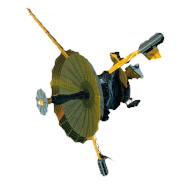GALILEO

## Galileo EPD Handbook

### Chapter 1. Instrument Summary

Figures: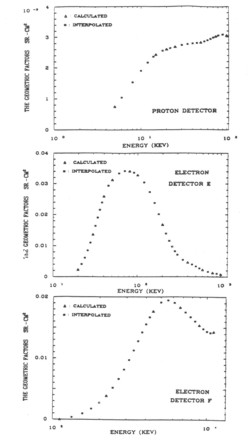Figure 1-59. The geometric factors of detectors of the Galileo LEMMS sensor.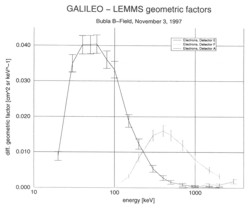Figure 1-60. LEMMS geometric factors.

Record Mode Rate Spectra: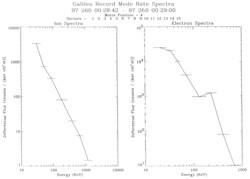Figure 1-61.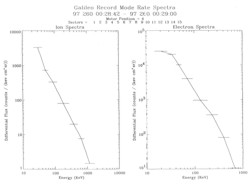Figure 1-62.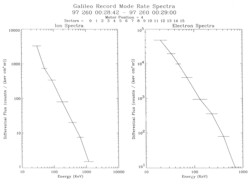Figure 1-63.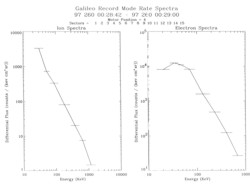Figure 1-64.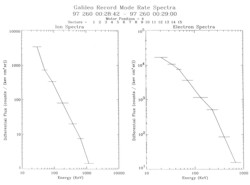Figure 1-65.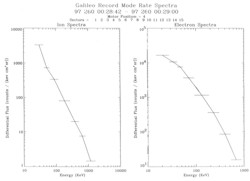Figure 1-66.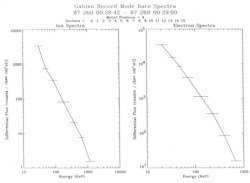Figure 1-67.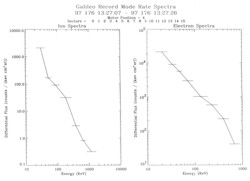Figure 1-68.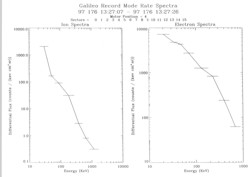Figure 1-69.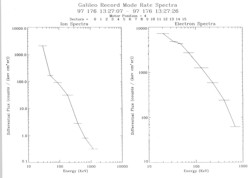Figure 1-70.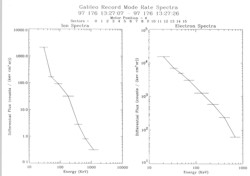Figure 1-71.